# 基于BP神经网络的小型四旋翼无人机的PID控制PID Control of Quadrotor UAV Based on BP Neural Network

DOI: 10.12677/OJAV.2020.81001, PDF, HTML, XML, 下载: 387  浏览: 2,006  国家自然科学基金支持

Abstract: The quadrotor UAV is a strong coupling and underactuated multi-degree of freedom nonlinear sys-tem, so the control of the quadrotor UAV is very important. This work optimizes the parameters of PID controller based on BP neural network. The results indicate that compared with the traditional PID control, the results from BP neural network have better performance, which would provide a new method and idea for the control design of small quadrotor UAV.

1. 引言

2. 四旋翼无人机系统的动力学建模

1) 四旋翼无人机机身不发生任何的形变，即认为无人机为刚体；

2) 在机体的质心上建立机体坐标系，整机质量均匀分布，质心在飞行过程中不发生偏移；

3) 在四旋翼无人机的飞行过程当中，重力加速度不随着飞行高度的变化而改变，保持一个定值。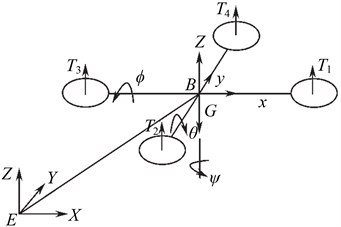Figure 1. Coordinate system of quadrotor UAV

$R=\left[\begin{array}{ccc}\mathrm{cos}\psi \mathrm{cos}\theta & \mathrm{sin}\theta \mathrm{sin}\phi \mathrm{cos}\psi -\mathrm{sin}\psi \mathrm{cos}\phi & \mathrm{sin}\theta \mathrm{cos}\phi \mathrm{cos}\psi +\mathrm{sin}\psi \mathrm{sin}\phi \\ \mathrm{sin}\psi \mathrm{cos}\theta & \mathrm{sin}\theta \mathrm{sin}\phi \mathrm{sin}\psi +\mathrm{cos}\phi \mathrm{cos}\psi & \mathrm{sin}\theta \mathrm{sin}\psi \mathrm{cos}\phi -\mathrm{sin}\phi \mathrm{cos}\psi \\ \mathrm{sin}\theta & \mathrm{sin}\phi \mathrm{cos}\theta & \mathrm{cos}\theta \mathrm{cos}\phi \end{array}\right]$ (1)

$G=mg$ (2)

${D}_{i}=\frac{1}{2}\rho {C}_{d}{\omega }_{i}^{2}={K}_{d}{\omega }_{i}^{2}$ (3)

${T}_{i}=\frac{1}{2}\rho {C}_{t}{\omega }_{i}^{2}={K}_{t}{\omega }_{i}^{2}$ (4)

${K}_{d}={C}_{d}\rho A{r}^{2}$ (5)

${K}_{t}={C}_{t}\rho A{r}^{2}$ (6)

$\left\{\begin{array}{l}\stackrel{¨}{x}=\left({F}_{x}-{K}_{1}\stackrel{˙}{x}\right)/m=\left[{K}_{t}{\sum }_{i=1}^{4}{\omega }_{i}^{2}\mathrm{cos}\psi \mathrm{sin}\theta \mathrm{cos}\phi +\mathrm{sin}\psi \mathrm{sin}\phi -{K}_{1}\stackrel{˙}{x}\right]/m\\ \stackrel{¨}{y}=\left({F}_{y}-{K}_{2}\stackrel{˙}{y}\right)/m=\left[{K}_{t}{\sum }_{i=1}^{4}{\omega }_{i}^{2}\left(\mathrm{sin}\psi \mathrm{sin}\theta \mathrm{cos}\phi -\mathrm{cos}\psi \mathrm{sin}\phi \right)-{K}_{2}\stackrel{˙}{y}\right]/m\\ \stackrel{¨}{z}=\left({F}_{z}-{K}_{3}\stackrel{˙}{z}-mg\right)/m=\left({K}_{t}{\sum }_{i=1}^{4}{\omega }_{i}^{2}\mathrm{cos}\theta \mathrm{cos}\phi -{K}_{3}\stackrel{˙}{z}\right)/m\end{array}$ (7)

$\left[\begin{array}{c}p\\ q\\ r\end{array}\right]=\left[\begin{array}{c}\stackrel{˙}{\phi }-\stackrel{˙}{\psi }\mathrm{sin}\theta \\ \stackrel{˙}{\theta }\mathrm{cos}\phi +\stackrel{˙}{\psi }\mathrm{sin}\phi \mathrm{cos}\theta \\ -\stackrel{˙}{\theta }\mathrm{sin}\phi +\stackrel{˙}{\psi }\mathrm{cos}\phi \mathrm{cos}\theta \end{array}\right]$ (8)

$\left[\begin{array}{c}\stackrel{˙}{\phi }\\ \stackrel{˙}{\theta }\\ \stackrel{˙}{\psi }\end{array}\right]=\left[\begin{array}{c}\left(p\mathrm{cos}\theta +q\mathrm{sin}\theta \mathrm{sin}\phi +r\mathrm{sin}\theta \mathrm{cos}\phi \right)/\mathrm{cos}\theta \\ q\mathrm{cos}\phi +r\mathrm{sin}\phi \\ \left(q\mathrm{sin}\phi +r\mathrm{cos}\phi \right)/\mathrm{cos}\theta \end{array}\right]$ (9)

$I=\left[\begin{array}{ccc}{I}_{x}& 0& 0\\ 0& {I}_{y}& 0\\ 0& 0& {I}_{z}\end{array}\right]$ (10)

$\left[\begin{array}{c}\stackrel{˙}{p}\\ \stackrel{˙}{q}\\ \stackrel{˙}{r}\end{array}\right]=\left[\begin{array}{c}\left[{M}_{x}+\left({I}_{x}-{I}_{z}\right)qr\right]/{I}_{x}\\ \left[{M}_{y}+\left({I}_{z}-{I}_{x}\right)rp\right]/{I}_{y}\\ \left[{M}_{z}+\left({I}_{x}-{I}_{y}\right)pq\right]/{I}_{z}\end{array}\right]$ (11)

$\left[\begin{array}{c}{U}_{1}\\ {U}_{2}\\ {U}_{3}\\ {U}_{4}\end{array}\right]=\left[\begin{array}{c}{K}_{t}{\sum }_{i=1}^{4}{\omega }_{i}^{2}\\ {K}_{t}\left({\omega }_{4}^{2}-{\omega }_{2}^{2}\right)\\ {K}_{t}\left({\omega }_{3}^{2}-{\omega }_{1}^{2}\right)\\ {K}_{t}\left({\omega }_{3}^{2}-{\omega }_{1}^{2}\right)\\ {K}_{d}\left(-{\omega }_{2}^{2}+{\omega }_{3}^{2}-{\omega }_{4}^{2}\right)\end{array}\right]$ (12)

1) 不考虑无人机与空气之间的摩擦，即忽略空气中的摩擦阻力；

2) 忽略无人机的螺旋桨的螺旋陀螺效应，则螺旋桨产生的升力和力矩与转速的平方有一定的线性关系；

3) 认为无人机的转动角度较小，因此绝对角速度 ${\left[p,q,r\right]}^{\text{T}}$ 可由欧拉角速度 ${\left[\stackrel{˙}{\phi },\stackrel{˙}{\theta },\stackrel{˙}{\psi }\right]}^{\text{T}}$ 代替。

$\left\{\begin{array}{l}\stackrel{¨}{x}=\left(\mathrm{cos}\phi \mathrm{sin}\theta \mathrm{cos}\psi +\mathrm{sin}\phi \mathrm{sin}\psi \right){U}_{1}/m\\ \stackrel{¨}{y}=\left(\mathrm{cos}\phi \mathrm{sin}\theta \mathrm{sin}\psi -\mathrm{sin}\phi \mathrm{cos}\psi \right){U}_{1}/m\\ \stackrel{¨}{z}=-g+\mathrm{cos}\phi \mathrm{cos}\theta {U}_{1}/m\\ \stackrel{¨}{\phi }=\stackrel{˙}{\theta }\stackrel{˙}{\psi }\left({I}_{y}-{I}_{z}\right)/{I}_{x}+l{U}_{2}/{I}_{x}\\ \stackrel{¨}{\theta }=\stackrel{˙}{\phi }\stackrel{˙}{\psi }\left({I}_{z}-{I}_{x}\right)/{I}_{y}+l{U}_{3}/{I}_{y}\\ \stackrel{¨}{\psi }=\stackrel{˙}{\phi }\stackrel{˙}{\theta }\left({I}_{x}-{I}_{y}\right)/{I}_{z}+{U}_{4}/{I}_{z}\end{array}$ (13)

3. 基于神经网络的PID控制器设计

PID控制参数的选取关系到控制效果的好坏，越精准的参数带来更优的控制效果。但是可以得到精确数值的理论计算整定法对于具有高度非线特点的四旋翼无人机模型显然适应性不强。而工程整定法虽然可以通过调试得到较好的控制效果的参数值，但是也存在着两个问题。第一，调试的效率较低。即使有着经验公式的帮助，参数的调试也不能一蹴而就，仍需要不断地试验。第二，调试的精度较差。要想在进一步的提升控制效果，单单靠不断地试验显然是不太现实的。因此工程整定法一般情况下不可能达到最优的参数设定。考虑到BP神经网络对于非线性函数出色的拟合能力，通过大量数据的训练，可以输出较为准确的参数。Table 1. The structure of the BP neural networkFigure 2. The training process of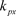under Adam optimizer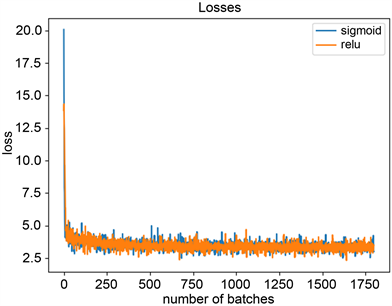Figure 3. The training process of ${k}_{px}$ under SGD optimizer

4. 算例Table 2. Performances of predictive values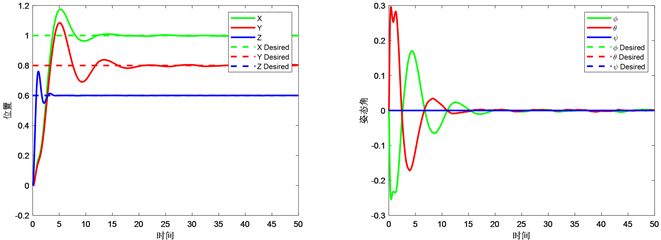Figure 4. Response curves under engineering setting method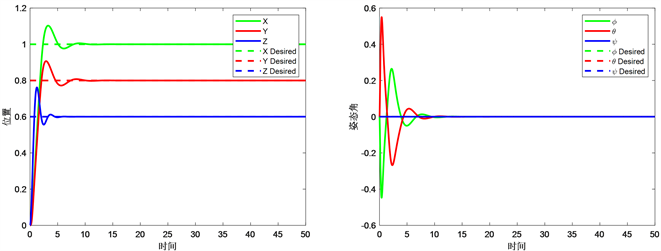Figure 5. Response curves under parameters predicted by BP neural network

5. 结论

1) 利用四旋翼无人机的动力学模型，进行数据的生成，通过生成的数据来训练网络可满足控制效果，在没有足够多的实验数据时，此方法不失为一种数据增强的有效方法。

2) 工程上通过经验所确定的PID控制器参数，传统的PID在无噪声的环境下可以使无人机到达指定的位置并保持稳定，但在有噪声的环境下，传统的PID控制器的表现并不是很好，即鲁棒性较差。

3) BP神经网络的预测能力能够有效地提高PID控制器的参数选取的精度，从而拥有更好的控制效果。

NOTES

*通讯作者。

  李德仁, 李明. 无人机遥感系统的研究进展与应用前景[J]. 武汉大学学报•信息科学版, 2014, 39(5): 505-513.  Park, S., Won, D.H., Kang, M.S., et al. (2005) RIC (Robust Internal-Loop Compensator) Based Flight Control of a Quad-Rotor Type UAV. IEEE International Conference on Intelligent Robots and Systems, Edmonton, 2-6 August 2005. https://doi.org/10.1109/iros.2005.1545113  Mian, A.A. and Wang, D. (2008) Nonlinear Flight Control Strategy for an Underactuated Quadrotor Aerial Robot. IEEE International Conference on Intelligent Robots and Systems, Sanya, 6-8 April 2008, 938-942. https://doi.org/10.1109/icnsc.2008.4525351  吴成富, 刘小齐, 袁旭. 四旋翼无人机建模及其PID控制律设计[J]. 电子设计工程, 2012(16): 74-76.  Rinaldi, F., Chiesa, S. and Quagliotti, F. (2012) Linear Quadratic Control for Quadrotors UAVs Dynamics and Formation Flight. Journal of Intelligent & Robotic Systems, 70, 203-220. https://doi.org/10.1007/s10846-012-9708-3  Lee, D., Kim, H.J. and Sastry, S. (2009) Feedback Linearization vs. Adaptive Sliding Mode Control for a Quadrotor Helicopter. International Journal of Control, Automation and Systems, 7, 419-428. https://doi.org/10.1007/s12555-009-0311-8  Das, A., Lewis, F. and Subbarao, K. (2009) Backstepping Approach for Controlling a Quadrotor Using Lagrange form Dynamics. Journal of Intelligent and Robotic Systems, 56, 127-151. https://doi.org/10.1007/s10846-009-9331-0  Efe, M.Ö. (2011) Neural Network Assisted Computationally Simple 〖PI〗^λ D^μ Control of a Quadrotor UAV. IEEE Transactions on Industrial Informatics, 7, 354-361. https://doi.org/10.1109/tii.2011.2123906  Muliadi, J. and Kusumoputro, B. (2018) Neural Network Control System of UAV Altitude Dynamics and Its Comparison with the PID Control System. Journal of Advanced Transportation, 2018, 1-18. https://doi.org/10.1155/2018/3823201